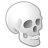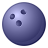# Grid overlay and spatial calculations help!

1155
2
03-23-2013 10:19 PMNew Contributor
Hi everyone,

I have a dataset that contains polygons over a large area with individual attribute values assigned to each, I want to:

- overlay a grid on top of the polygons layer

- calculate a value based on the individual value each polygon is assigned and the percentage of coverage it has within each cell
i.e.

polygon 1 = 3.3
polygon 2 = 2.2
polygon 3 = 1.5

polygon 1 = 25% coverage within cell 1,1
polygon 2 = 25% coverage within cell 1,1
polygon 3 = 50% coverage within cell 1,1

value of cell 1,1

3.3/100 * 25 = .825
2.2/100 * 25 = .55
1.5/100 * 50 = .75        =      .825 + .55 + .75 = 2.125

- Assign the calculated value to that grid cell.

If anyone could give me some input into how i can go about doing this it would be much appreciated!

Thanks!
Tags (2)
2 RepliesOccasional Contributor III
Hi Richard,

You can do this by using a combination of the Intersect Tool, Spatial Join Tool, and Field Calculations.

1. Run the intersect tool with the polygons and grid as the inputs
2. Add a field called poly_area, calculate geometry to get the area of the intersections
3. Run the Spatial Join tool to attach the area of the Grid feature class to the output of the intersect tool
4. Add a field called percentage, calculate this to be poly_area/grid_area
5. Add a field called val_percent, calculate this to be your value field * percentage
6. Run the Spatial Join tool again to attach val_percent to the grid, in the Field Mapping, right click val_percent and select the merge rule of SUM

Best,
Melanie S.New Contributor
Thank you very much Melanie!